## Solution to 1986 Problem 75

 We use Kepler's Third Law, which states that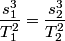\begin{align*}\frac{s_1^3}{T_1^2} = \frac{s_2^3}{T_2^2}\end{align*}for any two bodies orbiting the same mass. Here, we let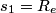$s_1 = R_e$ be the radius of the earth,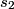$s_2$ be the radius of the synchronous satellite orbit,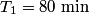$T_1 = 80 \mbox{ min}$ be the period of the hypothetical satellite orbiting at sea level, and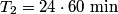$T_2 = 24 \cdot 60 \mbox{ min}$ be the period of the synchronous satellite. Then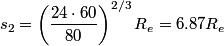\begin{align*}s_2 = \left(\frac{24 \cdot 60}{80}\right)^{2/3} R_e = 6.87 R_e\end{align*}Thus, answer (B) is correct.